telescopeѲptics.net          ▪▪▪▪                                             CONTENTS

# 4.1.2. Lower-order spherical aberration: aberration function, blur size

The P-V wavefront error of primary spherical at paraxial focus is W=s(ρd)4, with s=0w40 being simplified notation for the aberration coefficient from Table 4. Wave aberration function for the lower-order, or primary spherical aberration, defining the P-V wavefront error as optical path difference at the best (diffraction) focus is:

Ws = sd4(ρ4-ρ2)  =  S(ρ4-ρ2)               (7)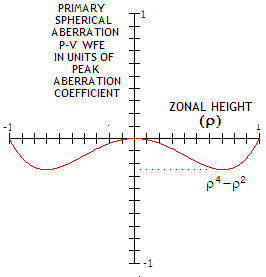with s being the aberration coefficient for spherical aberration, d the pupil radius and ρ the ray height at the pupil in units of the pupil radius. The quantity S=sd4 is the peak aberration coefficient, equal to the P-V wavefront error at paraxial focus. Quantity in the brackets determines the aberration maximum at the best (diffraction) focus in terms of the peak aberration coefficient as S/4 for ρ=0.5. Graph at left shows the form of the aberration function for unit peak aberration coefficient, i.e. ρ4-ρ2.

The sign of P-V wavefront aberration with respect to reference sphere centered at paraxial focus is negative, its numerical value equaling the aberration coefficient, while positive with respect to the reference sphere centered at the best focus (based on the aberration being positive when the path length from the aberrated point is longer, and negative when it is shorter than path length from the corresponding point at the reference sphere).

You will notice that this function form for spherical aberration differs from the one given for primary aberrations (Eq. 5.1). This is because Eq. 5.1 gives the aberration function for paraxial focus, or so-called classical aberrations. Advance in calculation methods revealed that Gaussian image point is not the best focus location for the three aberrations affecting point-image quality - spherical, coma and astigmatism. Each of them requires shift from the Gaussian image point to their respective best focus location, where the central intensity of diffraction pattern is at its maximum (thus, best focus location is also called diffraction focus). Primary aberrations evaluated at the best focus location are called orthogonal or balanced primary aberrations.

For spherical aberration, the amount of defocus from paraxial focus needed for the shift to diffraction focus location is given by P=-S, with P and S being the peak aberration coefficients for defocus and spherical aberration, respectively (FIG. 36). Since for identical longitudinal aberration the P-V wavefront error of defocus is double the P-V error of spherical aberration at paraxial or marginal focus (or P=-2S for the entire longitudinal spherical aberration), best focus for spherical aberration is at the mid point of its longitudinal aberration, as already stated. Both peak aberration coefficients equal the P-V wavefront error, as Wd=Pρ2 and WsP=Sρ4 (the peak aberration coefficient S equals the P-V error at the paraxial focus), so the combined aberration is Ws=S+P=Ws+Wd=Sρ4+Pρ2 which, for P=-S can be written as Ws=S(ρ42).

Likewise, the P-V wavefront error at the marginal focus and the focus of minimum geometric blur is Ws=S(ρ4-2ρ2) and Ws=S(ρ4-1.5ρ2), respectively (FIG. 33 right).

Another difference between the classical and best focus primary spherical aberration is in the sign of the P-V wavefront error. With one of the two forms of the classical spherical aberration - under-correction at the paraxial focus - the wavefront point of maximum aberration lies closer to the focus than the corresponding reference sphere point (that is, wave from the aberrated point has smaller optical path length), it is of negative sign (FIG. 33 left). On the other hand, the point of maximum P-V deviation for balanced (best focus) spherical aberration is farther away than its perfect reference point, giving to the wavefront deviation positive sign. Hence, the aberration coefficient for spherical mirror is negative for the classical aberration form, and positive for the best focus aberration form. It reverses in the case of over-correction.

FIG. 36A shows the basic properties of spherical aberration at four points of its longitudinal aberration: paraxial, best (minimum wavefront deviation) focus, smallest geometric blur focus, and marginal focus.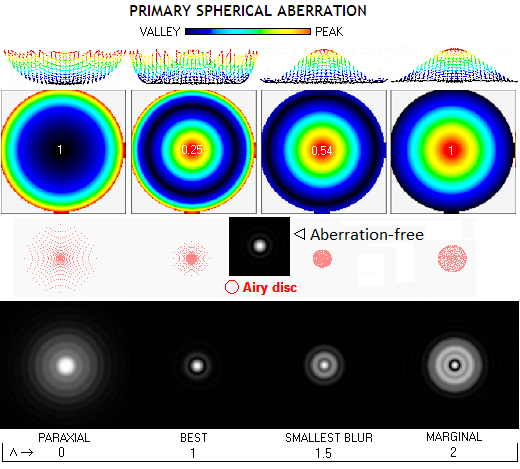FIGURE 36A: Top to bottom: 3-D wavefront deviation plot, wavefront map, ray spot diagrams and actual diffraction patterns for 1/4 wave P-V of spherical aberration at the best focus (Λ=1), paraxial (Λ=0), marginal (Λ=2) and 0.866 zone focus (Λ=1.5, circle of least confusion). Here, the perfect pattern has faint, but visible rings(1). They grow noticeably brighter due to the energy spread by the aberration. The relation between the geometric blur size and the appearance of diffraction pattern is rather loose: while the smallest geometric blur (Λ=1.5) is half the blur at the best focus location (Λ=1), which is 8/2.44 Airy discs in diameter, diffraction effect for the former is significantly worse. Ray density gives better indication of energy scatter than the blur size itself. Note that the 3D plots on top do not show the actual relative heights, which are in proportion to the P-V value on the wavefront map below.

(1) Bright stars will display more pronounced diffraction rings, especially the first, which may appear nearly as bright as the central disc even with near perfect (aberration-free, unobstructed) pattern. This is due to a logarithmic intensity response of the eye: a 56 times lower intensity of the first bright ring may appear to the eye less than twice fainter. Only as the first ring intensity drops close to the threshold of perception, the central disc begin to appear much brighter.

The above best focus concept is valid for relatively small P-V wavefront errors. While the P-V/RMS wavefront error always remain the lowest at the best focus location, diffraction peak shifts away from it for errors larger than 0.625 wave P-V. At this error level, diffraction peak evens up over 40% of the longitudinal aberration centered around best focus. With larger errors, two diffraction peaks form, one closer to paraxial focus, and one farther away than the best focus. Peak diffraction intensity is still higher at the best focus than either paraxial or marginal, it still has the lowest P-V/RMS error, but since diffraction peak has shifted away from it, it is not the best (diffraction) focus. Plots below show axial intensity distribution for selected values of the peak aberration coefficient.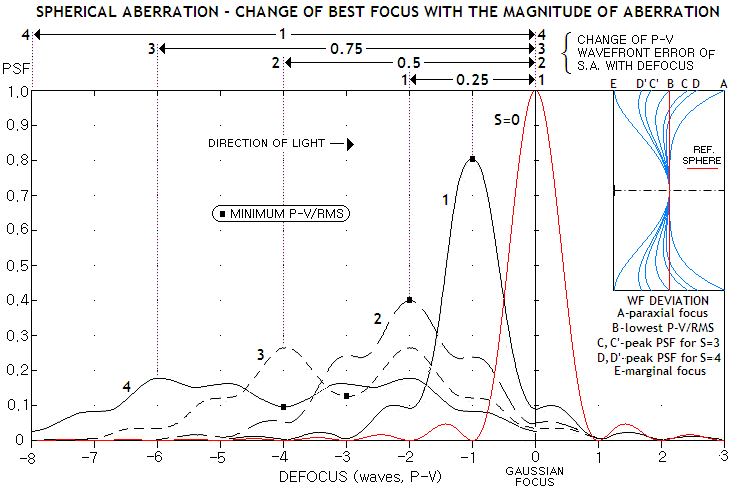FIGURE 36B: Axial longitudinal diffraction intensity distribution for aberration free point image (red) and four values of the peak aberration coefficient (which, expressed in units of wavelength, equals the P-V wavefront error at the paraxial, or Gaussian focus). The negative (i.e. toward objective) spread of energy indicates under-correction; the plots would mirror-reverse around Gaussian focus for overcorrection. For S=1 and S=2, best (diffraction) focus coincides with the mid focus point, where the defocus peak coefficient equals S (i.e. defocus P-V wavefront error equals the P-V wavefront error of spherical aberration at the paraxial focus, opposite in sign), and the P-V/RMS wavefront error is the lowest, four times smaller than at the paraxial (or marginal) focus. For S=3 and S=4, however, PSF peak is not at the point with the minimum wavefront error (WFE), but at the points 0.666/1.333 and 0.5 and 1.5 on the longitudinal aberration length (distance between paraxial and marginal foci - equaling twice as many waves of defocus as the P-V error at paraxial focus - normalized to 2). Inset top right shows profiles of the wavefront with their relative depths (P-V errors). Plots below illustrate PSF and MTF for the minimum P-V/RMS wavefront error focus, and for the two PSF peaks
for S=3, i.e. 0.75
λ P-V wavefront error at the focus of minimum wavefront deviation, midway between paraxial and marginal focus. With the defocus P-V for given LA being twice the P-V of spherical aberration at paraxial focus, the corresponding defocus P-V WFE is 6 waves, and the defocus from the mid focus - the one with the lowest P-V/RMS error, at -3 waves of defocus from paraxial focus - to the foci with the highest PSF peak (Strehl) at -2 and -4 waved of defocus, respectively, are shifted by +λ and -λ from it (plots generated by Aberrator).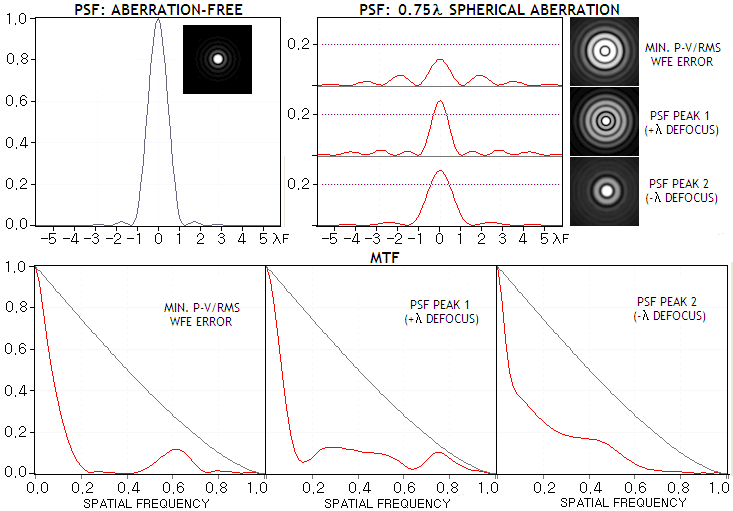The two PSF peaks have identical Strehl, but different intensity distributions and, consequently, different contrast transfer properties. For S=4 (hence 1 wave P-V WFE at the mid focus) for instance, PSF maximum is at the defocus values of 0.5 and 1.5 of the 8 waves long LA
normalized to 2 (thus, equaling 2 and 6 waves of defocus, respectively), where the wavefront profile is given by ρ4-0.5ρ2 and ρ4-1.5ρ2, respectively. The corresponding relative P-V wavefront error is 0.5625 for both, the former (a sum of the absolute values of the profile equation for the normalized zonal height ρ values of ρ=1 and ρ=0.5), and latter (profile equation for ρ=0.866), and larger by a factor 2.25 from the P-V wavefront error at mid focus (given by ρ42 for ρ=0.51/2). Despite more than twice lower P-V/RMS error at the mid focus, its PSF peak is almost twice lower.
In the presence of central obstruction, a larger error magnitude is needed to result in the shift of PSF peak away from the point of minimum wavefront deviation. For 50% linear obstruction, the shift starts taking place as the error at the mid focus exceeds 1 wave P-V (at about 0.9 waves P-V peak PSF intensity flattens axially around mid focus, remaining nearly unchanged over more than 1/5 of the LA length).  For smaller obstructions, the difference vs. clear aperture quickly diminishes; with 33% linear obstruction and S=3, axial PSF intensity develops low at the mid focus and two peaks before and after it, similar to the one shown for clear aperture above, only with the low about half as deep.

The reason for these seemingly illogical results is that the phase error - which determines the diffraction effect - is, unlike the wavefront error, cyclical. The resulting amplitude from two interfering waves diminishes from 2 to 0 as the optical path difference (OPD) increases from 0 to 0.5 waves. Then it increases from 0 to 2 as OPD further increases from 0.5 to 1 wave (full wave phase difference results in the two waves interfering in phase), and so on. It is the profile of the wavefront which ultimately determines the phase sum at any one point, and the corresponding PSF.

Calculating the aberration coefficient

In order to obtain specific wavefront aberration, we need to calculate the aberration coefficient s. General expression for the aberration coefficient of spherical aberration of a spherical thin lens is: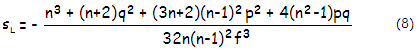with n being the refractive index, q=(R2+R1)/(R2-R1) the lens shape factor, and p=1-2f/I the lens position factor (f is the lens focal length, and I the image-to-lens separation).

For object at infinity, f=I and p=-1, and

sL=-[n3+(n+2)q2+(3n+2)(n-1)2-4(n2+1)q]/32n(n-1)2f3.

For equi-convex or equi-concave lens, q=0 and it becomes:

sL=-[n3+(3n+2)(n-1)2]/32n(n-1)2f3.

For plano-concave or plano-convex lens facing incoming light with the curved surface, q=1 and

sL=-[n3+(n+2)+(3n+2)(n-1)2-4(n2-1)]/32n(n-1)2f3.

When facing light with the flat surface, q=-1 and sL=-[n3+(n+2)+(3n+2)(n-1)2+4(n2-1)]/32n(n-1)2f3.

Substituting n=1.5 for crown-like glass, gives sL=-1/1.74545f3, sL=-1/3.42857f3, and sL=-1/0.88888f3 for the three, respectively.

In other words, for given aperture and focal length, an equi-concave thin lens will generate nearly twice more of primary spherical aberration than plano-concave lens facing incoming light with its curved surface, and only half as much than plano-concave lens facing incoming light with its flat surface.

Making one of lens surfaces aspheric of conic K results in a change in the aberration coefficient given as:

sLa=sL+(n-n')K/8R3.

It doesn't affect other aberrations, including chromatic.

Suffice to say, single spherical lens cannot be free from spherical aberration, except for the object inside the focal point of a positive or negative meniscus with specific values of p and q (in other words, lens forming a real image cannot have spherical aberration cancelled). The aberration is at its minimum for q=-2p(n2-1)/(n+2). Aspherizing lens surface into conic K<0 (ellipsoid, paraboloid, hyperboloid) for given center thickness increases the in-glass path toward the edge (the conic surface being shallower than sphere). This induces overcorrection, given as the P-V error at the best focus for a single surface by W=(n'-n)Kd4/32R3, with d being the lens aperture radius and R the surface radius of curvature. More detailed evaluation of the lens primary spherical aberration is given in section on spherochromatism.

Fortunately, calculating the aberration coefficient for mirror surface is quite simple. Its general form is given by: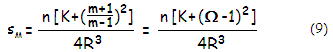with n being the index of refraction of incidence medium, K being the mirror conic, m the magnification, R the radius of curvature and Ω=R/O the inverse of the object distance O in units of mirror's radius of curvature, numerically positive.

Note that the above relation for the coefficient is derived from the general surface coefficient for spherical aberration, using J=(m+1)/(m-1)R and N=-2/nR, valid for mirror surface.

Optical magnification, defined as the ratio of image-to-object size, thus numerically negative when image orientation is opposite to that of the object. For object to the left, and image to the right of the aperture stop it is given by m=(I/O), I and O being for the image (numerically positive) and object distance (numerically negative) from the objective. For the object distance O known it is obtained from m=f/(O+f) when the focal length f is positive, and m=-f/(O-f) for negative focal length. If we denote the reciprocal of the object distance in units of the mirror focal length (numerically negative) as ψ=f/O, then the magnification is m=ψ/(ψ-1), and the aberration coefficient is: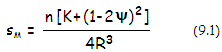Setting the sum in brackets to zero defines both, zero-aberration conic for given object distance as K=-(1-2ψ)2 and zero-aberration distance for given conic - after expanding (1-2ψ)2 and solving for ψ - as O=2f/(1-f-K), for the object at the far (+), and near focus (-) of the conic. Object distance for K>0 is not defined in this form, because the foci of the oblate ellipse (with respect to horizontal axis) lie off axis.

For distant objects (in astronomy, practically at infinity) m=0 and the coefficient reduces to: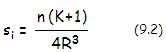This gives the peak aberration coefficient for mirror surface and object at infinity as: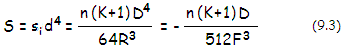with F being the mirror focal ratio, given by -f/D (for mirror in air oriented to the left, f<0 and n=1).

The P-V wavefront error of spherical aberration at the paraxial focus equals the peak aberration coefficient, Wsp=S, and the corresponding RMS wavefront error ωsp is given as ωsp=Wsp/1.5√5. As mentioned, the P-V wavefront error at the paraxial focus is larger than the P-V error at the best (diffraction) focus by a factor of 4. Since the P-V to RMS wavefront error ratio remains unchanged, the RMS wavefront error at the best focus is ωsb=Wsp/√180 in terms of the P-V wavefront error at the paraxial, and ωsb=Wsb/1.5√5 in terms of the best focus P-V error.

The P-V and RMS wavefront error in units of the wavelength are related to the relative blur diameter at the best focus, expressed in units of Airy disc diameter, as Bsb=32Wsb/2.44 and Bsb=12√5ωsb/2.44, or Bsb=8√180ωsb/2.44, respectively (with Wsb being the P-V wavefront error at the best focus in units of the wavelength for which the Airy disc is calculated). For Wsb=0.25, the 0.80 Strehl level, it gives blur diameter of 3.28 Airy disc diameters. As mentioned, the blur is twice as large at the paraxial focus location, while the smallest blur, at 3/4 of the longitudinal aberration from paraxial focus, is twice smaller.

The significance of the medium refractive index n is that it determines the nominal wavelength and, with it, the size of a given wavefront error relative to it. In slower media, such as glass, light waves are in effect compressed, and a given nominal wavefront error results in a proportionally greater phase difference at the focus. From another perspective, the compressed wavelength results in smaller Airy disc, while the size of transverse aberration (blur) remains unchanged, thus making the actual aberration proportionally larger.

Spherical aberration can also be expressed in terms of the peak aberration coefficient S as ray aberrations:

L = 16SF2ρ2,        T = 16SFρ3     and      Ta = 16Sρ3/D         (10)

for the longitudinal, transverse and angular aberration (in radians), respectively, with D being the pupil diameter, and 0<ρ≤1 the relative ray height in the pupil with the radius normalized to 1. Their respective aberration maximums, for ρ=1 and object at infinity, are given with: L=(1+K)D/32F, T=(1+K)D/32F2 and Ta=(1+K)/32F3. The ρ parameter shows that longitudinal spherical aberration changes with the square of the ray height, while transverse and angular aberration change with the cube of ray height in the pupil. The transverse and angular aberration are for the blur diameter at the paraxial focus; blur at the best focus location is smaller by a factor of 0.5, and the circle of least confusion by a factor of 0.25.

From the relation for longitudinal aberration in Eq. 10, the best focus P-V wavefront error in terms of longitudinal aberration and focal ratio is given by Ws=S/4=L/64F2 (for ρ=1). In units of 550nm wavelength, it is Ws=28.4L/F2.

The sign of peak aberration coefficient determines the sign of ray aberration as negative, indicating that marginal ray from the mirror, determining blur radius, focuses shorter, thus intersecting the blur plane below the axis (this also holds for best focus location, but not for the marginal focus location, where the blur boundary is formed by converging rays - that is, rays that focus farther away from the image plane - and all three ray aberrations are positive).

What could be of interest is the RMS blur radius for various locations within the span of longitudinal spherical aberration. It can also be expressed in terms of normalized longitudinal aberration Λ, and peak aberration coefficient S, as: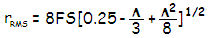In units of the paraxial blur radius, the RMS blur radius is just the square root of the quantity in brackets, [0.25-(Λ/3)+(Λ2/8)]1/2. Location of the smallest RMS blur radius is found at Λ=4/3 (defocus location between best focus and circle of least confusion), where it is smaller than (geometric) paraxial blur radius by a factor of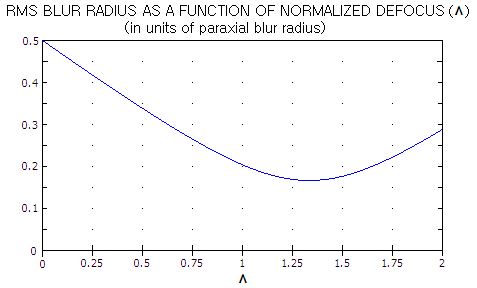0.167 (in comparison, the best focus and circle of least confusion RMS blur radii are smaller than paraxial blur radius by a factor of 0.204 and 0.177, respectively). Knowing that the geometric blur radius for Λ=0 (paraxial focus), 1 (best focus), 1.5 (smallest blur) and 2 (marginal focus) is 1, 0.5, 0.25 and 0.385 - also in units of the paraxial blur radius - respectively, we see that the ratio RMS-to-geometric blur is significantly smaller for the best and paraxial foci than for the other two.

The relative wavefront aberration for Λ=4/3 is ŵ=0.408 (Eq. 6), which is smaller than that at the location of the circle of least confusion (ŵ=0.545), but still significantly higher than at the best focus location (ŵ=0.25). It shows that the RMS ray blur size, while generally somewhat more meaningful than the geometric ray blur size, still lacks the accuracy required of a reliable indicator of optical quality.

In units of the Airy disc diameter, the RMS blur diameter is RRMS=8S[0.25-(Λ/3)+(Λ2/8)]1/2/1.22, for the peak aberration coefficient S in units of the wavelength. Since the P-V error at the best focus Ws=S/4, it can also be written as RRMS=2Ws[0.25-(Λ/3)+(Λ2/8)]1/2/1.22 which, with Λ=1 at for best focus location, becomes RRMS=16Ws/1.226.

In terms of the RMS wavefront error ωs, RRMS=430ωs/1.22

EXAMPLE: Running all the numbers for a 6" f/8.15 sphere, thus F=8.15, with d=3" and R=-97.8", gives the aberration coefficient for object at infinity s=(1/4R3)=-0.000000267, the peak aberration coefficient S=sd4=-0.000021647, longitudinal aberration L=16SF2=D/32F=0.02298", and the RMS wavefront error ω=S/180=0.000001613". Expressing the peak aberration coefficient - which equals the P-V wavefront error at the paraxial focus - in units of 550nm wavelength (0.00002165"), gives the P-V wavefront error at the paraxial focus of 1 wave, the P-V wavefront error at the best focus of 1/4 wave (for ρ=0.5), and the best focus RMS wavefront error of 1/13.4 wave.

The transverse blur diameter at the best focus, T=8SF=D/64F2 or, in Airy disc diameters, is Bs=8S/2.44λ=3.28 is half the blur diameter at the paraxial focus, and twice the smallest blur diameter (circle of least confusion). Angular blur diameter at the best focus Ta=T/f=1/64F3=8S/D=0.00002886 radians, or 0.00002886x206,265=5.95 arc seconds. Since both, wavefront error and geometric (ray) aberrations are directly proportional to the aberration coefficient, it implies that they are in a constant proportion themselves. In other words, doubling the wavefront error also doubles the geometric aberration.

The RMS blur radius at the best focus (Λ=1) is rRMS=8FS[0.25-(Λ/3)+(Λ2/8)]1/2=0.000288, and the RMS blur diameter in units of the Airy disc diameter RRMS=8S[0.25-(Λ/3)+(Λ2/8)]1/2/1.22=1.338, for S in units of the wavelength.

For object at infinity, spherical aberration in either wavefront or ray form, is independent of the position of aperture stop. That makes it relatively simple to find out the combined spherical aberration coefficient for two or more mirrors. For a pair of mirrors, the combined peak aberration coefficient is given by Sc= S1 + S2, (Eq. 9), with the aperture diameter D for the second surface being determined by the relative height k of marginal ray at it, in units of the primary mirror semi-diameter (equal to the minimum secondary diameter in units of the primary diameter). Since the object for the second surface is the object-image formed by the preceding surface, magnification m for the second surface is greater than zero, given by m=R2/(R2-kR1), R1 and R2 being the radii of curvature of the first and second surface, respectively.